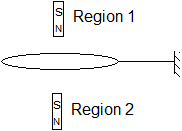# Doubt from Magnetism

.

There is a circular loop which is fixed in horizontal plane. Magnet is released then the acceleration of magnet is less than the gravity(g) inAnswer : In both Region 1 and 2 .Explain how?

This is a direct consequence of Lenz's Law. You will find this useful:

When magnet will be in region 1 then loop will act as N pole and hence will repel the magnet and hence it’s acc will be less than g
In region 2 again loop acts as N pole and hence attracts the S pole of magnet and hence will reduce it’s acc and thus it will be less than g